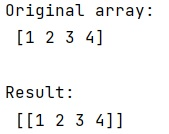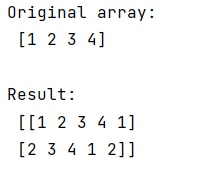# Difference Between reshape() and resize() Method in NumPy

NumPy reshape() vs. resize(): In this tutorial, we will learn about the difference between reshape() and resize() method in NumPy. By Pranit Sharma Last updated : April 10, 2023

## Difference Between reshape() and resize()

The differences between reshape() and resize() method is that:

• The numpy.reshape() is used to give a new shape to an array without changing its data whereas numpy.resize() is used to return a new array with the specified shape.
• The reshape() does not change our data, but resize() does.
• The resize() first accommodates all the values in the original array. After that, if extra space is there (or the size of the new array is greater than the original array), it adds its values.
• Note: reshape() will always try to return a view wherever possible, otherwise, it would return a copy.

Let us understand with the help of an example,

## Examples to demonstrate the difference between reshape() and resize() method in NumPy

Consider the below two examples to understand the difference between reshape() and resize() method in NumPy.

### 1) Example of reshape() Method

```# Import numpy
import numpy as np

# Creating an array
arr = np.array([1, 2, 3, 4])

# Display array
print("Original array:\n",arr,"\n")

# reshape
arr = arr.reshape(1, 4)

# Display result
print("Result:\n",arr,"\n")
```

#### Output### 2) Example of resize() Method

```# Import numpy
import numpy as np

# Creating an array
arr = np.array([1, 2, 3, 4])

# Display array
print("Original array:\n",arr,"\n")

# resize
arr = np.resize(arr, (2,5))

# Display result
print("Result:\n",arr,"\n")
```

#### Output